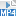## Content

### Antiderivatives of exponential and logarithmic functions

We've seen various derivatives so far, including

$\dfrac{d}{dx}\, e^x = e^x \qquad \text{and, more generally,} \qquad \dfrac{d}{dx}\, e^{kx} = k \, e^{kx},$ where $$k$$ is any non-zero real constant. Also, we've seen $\dfrac{d}{dx}\, \log_e x = \dfrac{1}{x} \qquad \text{and, more generally,} \qquad \dfrac{d}{dx}\, \log_e(ax+b) = \dfrac{a}{ax+b},$ for $$ax+b>0$$, where $$a,b$$ are real constants with $$a \ne 0$$. From this we can deduce several antiderivatives.

The basic indefinite integrals are

$\int e^x \; dx = e^x + c \qquad \text{and} \qquad \int \dfrac{1}{x} \; dx = \log_e x + c$ and, more generally, $\int e^{kx} \; dx = \dfrac{1}{k} \, e^{kx} + c \qquad \text{and} \qquad \int \dfrac{1}{ax+b} \; dx = \dfrac{1}{a} \, \log_e(ax+b) + c,$ where $$c$$ as usual is a constant of integration.

We can use these antiderivatives to evaluate definite integrals.

#### Example

Find

$\int_e^{e^2} \dfrac{1}{x} \; dx.$

#### Solution

\begin{align*} \int_e^{e^2} \dfrac{1}{x} \; dx &= \bigl[ \log_e x \bigr]_e^{e^2}\\ &= \log_e (e^2) - \log_e e = 2-1 = 1 \end{align*}

Exercise 11

Prove that, for any $$x>0$$,

$\int_1^x \dfrac{1}{t} \; dt = \log_e x.$

Exercise 12

Prove that, for any $$x>0$$ and $$n>0$$,

$\int_{x^n}^{x^{n+1}} \dfrac{1}{t} \; dt = \log_e x.$

Exercise 13

Differentiate $$f(x) = x \log_e x - x$$. Hence find the indefinite integral

$\int \log_e x \; dx.$

Screencast of exercise 13Warning! As we mentioned previously, $$\log_e x$$ is only defined for $$x>0$$, while $$\dfrac{1}{x}$$ is defined for all $$x \ne 0$$. So the equation $\int \dfrac{1}{x} \; dx = \log_e x + c$ is valid only for $$x>0$$ and, more generally, the equation $\int \dfrac{1}{ax+b} \; dx = \dfrac{1}{a} \, \log_e (ax+b) + c$ is valid only when $$ax+b>0$$. Although it is more complicated, it is sometimes necessary to consider the function $$\log_e|x|$$, which is defined for all $$x \neq 0$$ and which also has derivative $$\dfrac{1}{x}$$. The equation $\int \dfrac{1}{x} \; dx = \log_e |x| + c$ is valid for all $$x \neq 0$$, and the equation $\int \dfrac{1}{ax+b} \; dx = \dfrac{1}{a} \log_e |ax+b| + c$ is valid for all $$x$$ such that $$ax+b \neq 0$$.

Next page - Content - Graphing exponential functions